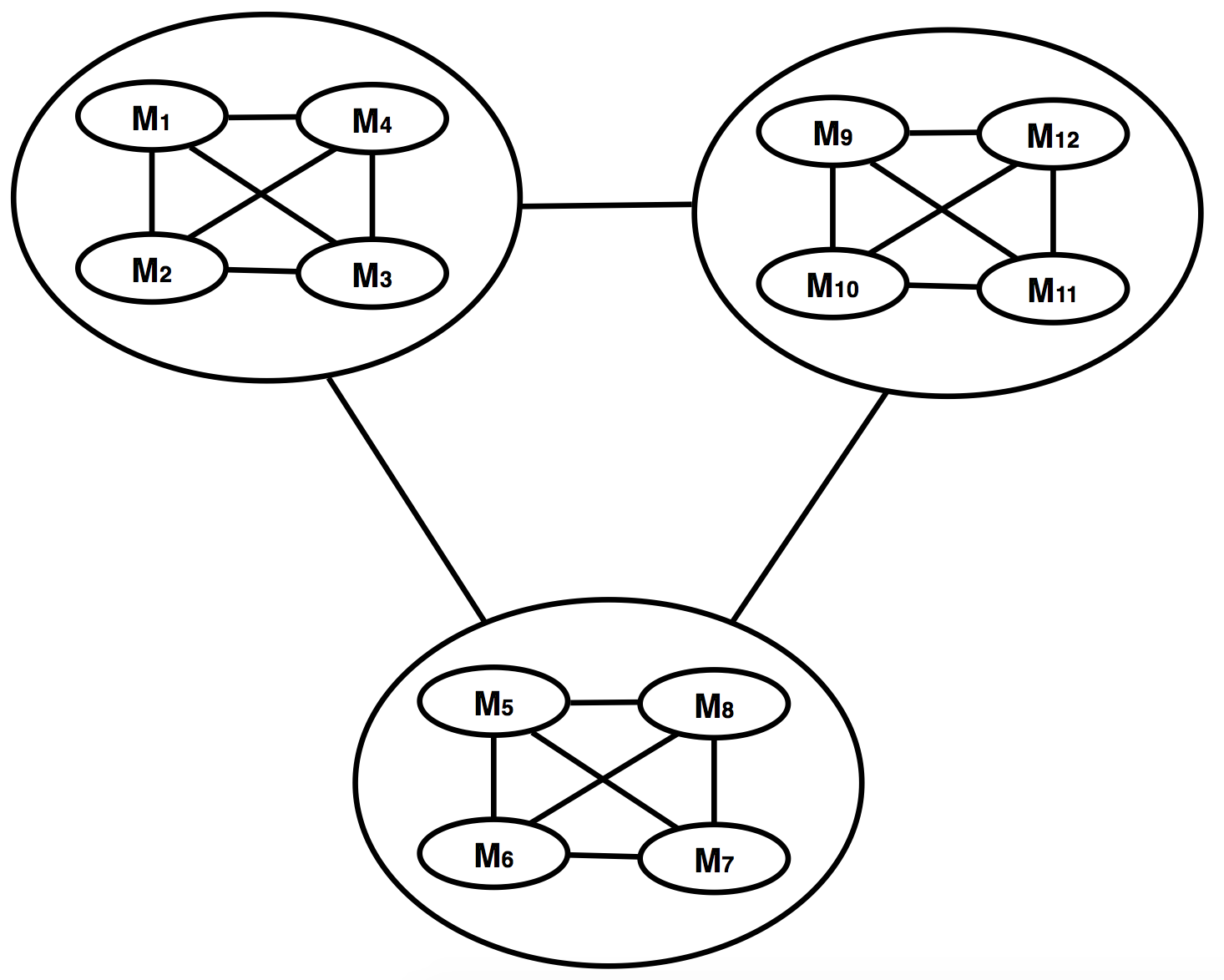## Tuesday 10 February 2015

### The effect of software topology in bug creation

In the last post, we implicitly assumed that we have a fully connected mesh topology in our software modules while there is almost no application in which all modules have a connection to all others.A not fully connected mesh topology
Although for example in the picture if you consider module M2 doesn't have any direct connection to M3, we can assume effects on M1 caused by M2 can has effect on M(via M1), but since we are building a simple model we ignore middle-men effects so we simply accept side neighbors effect at the moment.

We have to find out how we can fix our model to support such a topology. The answer is: WE CAN'T unless we have a model for our software. So let us build a general model for the software.

OK, suppose there is a restriction in the application that any 4 small module can have full mesh connection and the whole application itself which contains many 4M units can have fully connected mesh topology for its 4Ms.A 12 modules application decided to 3 fully connected mesh named as 4M and  then a higher level of fully connected mesh for each 4Ms.
For example for a 12 modules application, we can have something like this picture. Now let us see if anything changes in our previously driven formulas or not.

From the previous post we had:

IDB1  =  ψ(n-1) (Σ E+ Σ Ni)   (1)
DDB=   ε Σ EΣ Ni    (2)

It shows the formula number (2) or direct development bugs will be the same because it only depends on new lines of code and changes, no matter how many modules we have or how they are connected to each other.

But for formula number (1) which is indirect development bugs, for each 4 small modules we have the following bug count:

IDB1  =  ψ(4-1) (Σ E+ Σ N)  = 3 ψ (Σ E+ Σ Ni)

Just remember the index 1 in all of the above is to show the evaluation of the formula at time t1 nothing else. We need it later to see what happens if we make repeated development during the time on software.

But if you look at the picture, we have another level of connection network which is also a fully connected mesh, so for this network the indirect development bugs will be:

IDB1  =  ψ (n/4-1) (Σ E+ Σ Ni)

So the total IDB will be the sum of the 2 above:

IDB1  =  3 ψ(Σ E+ Σ Ni) + ψ(n/4-1) (Σ E+ Σ Ni)

or

IDB1  =  ψ (n/4+2) (Σ E+ Σ Ni)    (5)

To compare 5 and 4 we need to see the behavior of terms (n-1) and (n/4+2), which is like the following:

0 < n < 4      (n-1) < (n/4+2)
n = 4            (n-1) = (n/4+2)
4 < n            (n-1) > (n/4+2)

What does it say?

"If you are working on an application which has many modules, you need to think about the module connection topology or you will build a platform to distribute bugs across the application."

Consider an application which has 100 modules, then the difference is 99 and 27 almost 4 to 1. We will talk about the better models and also try to have a more accurate calculation later.